# Lambda decay, momentum of the pion and proton

## Homework Statement

I have a lambda decaying into a pion and a proton. The lambda is moving with velocity 0.9c and I know the mass of the lambda as well as the pion and proton (these are known constants). I need to find the momentum of the pion and the proton after the decay happens.

## Homework Equations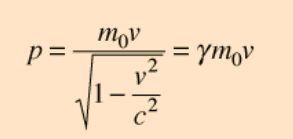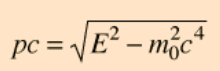Conservation of momentum and conservation of energy

## The Attempt at a Solution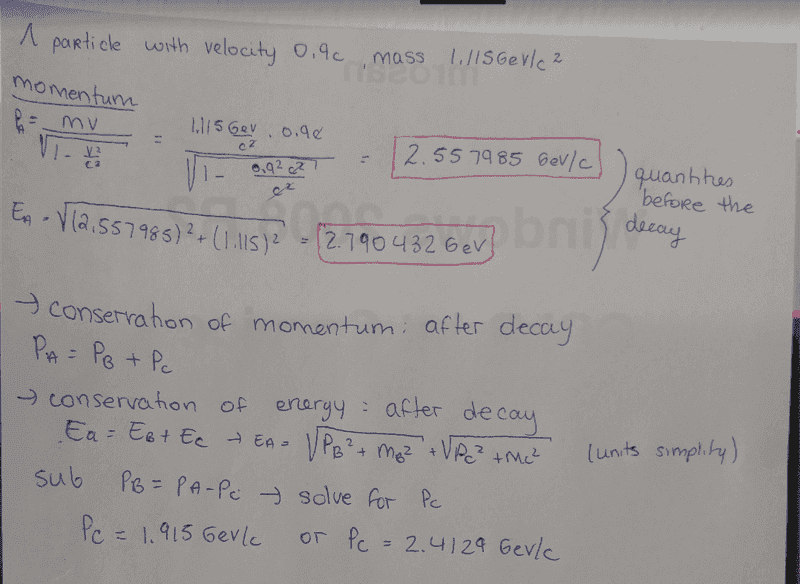I don't know at this point which momentum to choose out of the two. I solved the math using a software so I would assume it is right.

#### Attachments

Orodruin
Staff Emeritus
Homework Helper
Gold Member
2021 Award

There are two solutions because I assume you solve kind of the quadratic equation (so + or - the square root)
But I am having trouble realizing which answer is "wrong" since none of them give anything negative

Orodruin
Staff Emeritus
Homework Helper
Gold Member
2021 Award
Why would either be wrong? What are the possible decays in the Lambda rest frame.

Edit: I suggest you do the computation in the Lambda rest frame first and then interpret your solutions there.

Edit: I suggest you do the computation in the Lambda rest frame first and then interpret your solutions there.
That's kind of the first problem I am working on, the lambda is at rest and I need to find the momentums of the other particles.
I end up getting that the momentum is equal to the mass, which seems wrong to me. They don't have the same mass and if the momentum of particle A (mother) is 0, then Pb=-Pc ... so this can't be right.

Orodruin
Staff Emeritus
Homework Helper
Gold Member
2021 Award
Please show your computations in the Lambda rest frame. No, I am not referring to the possible decay channels, I am referring to the possible momenta of this particular decay.

Please show your computations in the Lambda rest frame. No, I am not referring to the possible decay channels, I am referring to the possible momenta of this particular decay.
https://imgur.com/rbiJNbT

In this page I tried kind of two different things and at one point got Pc=mc and then the other got a factor of sqrt2/2

Orodruin
Staff Emeritus
Homework Helper
Gold Member
2021 Award
I am sorry, but it is impossible to tell what you have tried to do from that photo. Please write it out here along with argumentation describing what you are doing and why.

I am sorry, but it is impossible to tell what you have tried to do from that photo. Please write it out here along with argumentation describing what you are doing and why.
Basically I was trying to apply this logic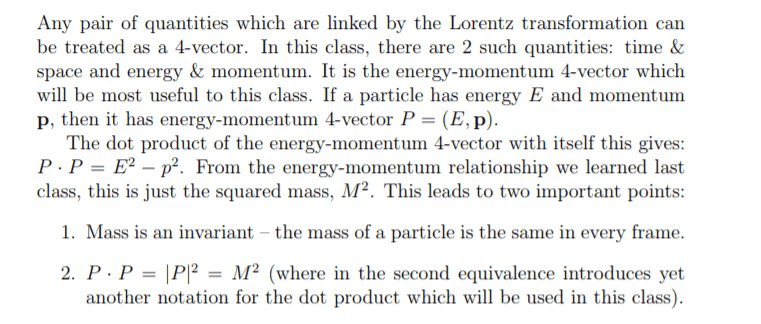Where I can write expressions for the momentum because it will be conserved, it will be a sum. Then I wanted to solve for an expression for the energy which I could equate to the more commonly known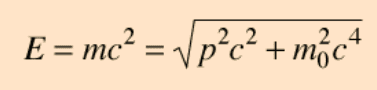And solve for the momentum, substituting as necessary since I can find an expression for Pa, and it is equal to 0 anyways

#### Attachments

Orodruin
Staff Emeritus
Homework Helper
Gold Member
2021 Award
I am sorry, it is still unclear. It is not clear from your handwriting what ##p##s represent 4-momenta and what ##p##s represent 3-momenta. Perhaps you are even mixing them up. This is why I suggest that you write down your entire reasoning again here, taking care to explain what you are doing in every step.

I am sorry, it is still unclear. It is not clear from your handwriting what ##p##s represent 4-momenta and what ##p##s represent 3-momenta. Perhaps you are even mixing them up. This is why I suggest that you write down your entire reasoning again here, taking care to explain what you are doing in every step.
This is the best I can do by myself. I haven't learned this stuff formally so trying to learn as I go, it's for a project and not even homework it will not be graded.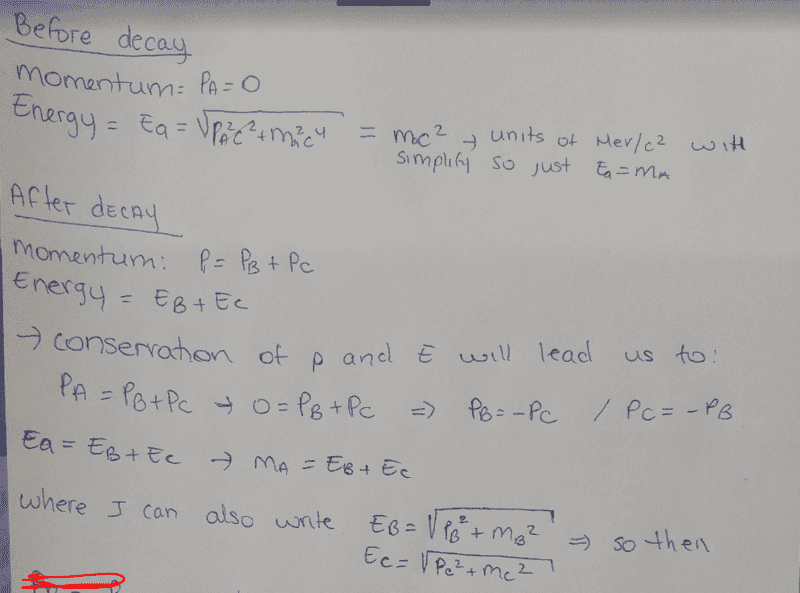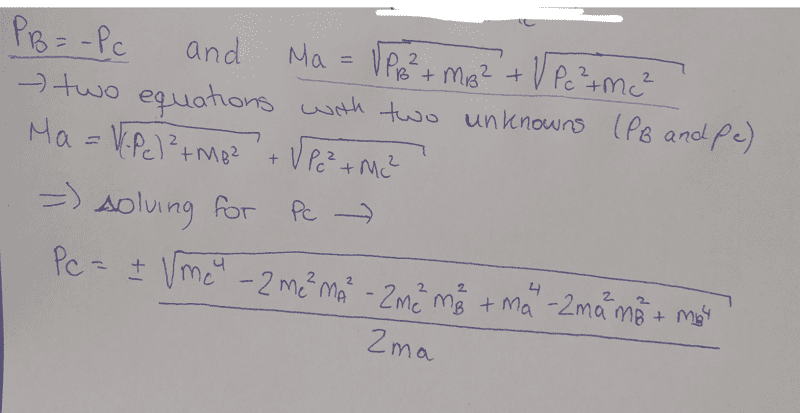It's just a lot of algebra and I thought maybe there was an easier way, there must be some concepts I just don't understand properly like the four vectors that I could apply here.

If I fill this in with the values of the lambda decay, I get that Pc=0.1485 Gev/c which is roughly the mass of the pion.

#### Attachments

Orodruin
Staff Emeritus
Homework Helper
Gold Member
2021 Award
If I fill this in with the values of the lambda decay, I get that Pc=0.1485 which is roughly the mass of the pion.
No, you get ##\pm## that.

No, you get ##\pm## that.
Yes, and Pb=-Pc.

I'm not sure what I'm missing out on here

Orodruin
Staff Emeritus
Homework Helper
Gold Member
2021 Award
How do you interpret the ##\pm##?

How do you interpret the ##\pm#?
Not sure, depending on the direction of travel along the x axis

Orodruin
Staff Emeritus
Homework Helper
Gold Member
2021 Award
Not sure, depending on the direction of travel along the x axis
So, are both solutions physical?

So, are both solutions physical?
Well I would think so. I assume that it can decay in any direction so the pion & proton are not forced to go "forwards"

Orodruin
Staff Emeritus
Homework Helper
Gold Member
2021 Award
Well I would think so. I assume that it can decay in any direction so the pion & proton are not forced to go "forwards"
So what does this tell you about the two solutions you found in the case of a moving ##\Lambda##?

So what does this tell you about the two solutions you found in the case of a moving ##\Lambda##?
then they are both possible. But I don't understand really, is my answer right? That the momentum would be + or - this whole expression (0.14)

So what does this tell you about the two solutions you found in the case of a moving ##\Lambda##?
The thing is, ultimately I am writing code to plot 10000 random lambda decays. I will plot the momentum and kinetic energy of the pion. So there aren't just 2 possibilities for the momentum. It will always depend on the angle at which it decays right ? Because 10 000 random decays could produce 10 000 different momentum right ?

Orodruin
Staff Emeritus
Homework Helper
Gold Member
2021 Award
The thing is, ultimately I am writing code to plot 10000 random lambda decays. I will plot the momentum and kinetic energy of the pion. So there aren't just 2 possibilities for the momentum. It will always depend on the angle at which it decays right ? Because 10 000 random decays could produce 10 000 different momentum right ?
Right, if you are looking at it in more than 1 space dimension you have a large distribution of momenta and energies depending on the angle. You do not need to write code to do this. What you need to do is to consider the decay isotropic in the rest frame of the ##\Lambda## and get the distribution on energy and momenta from there. You will find that the resulting decay products are emitted preferentially in the forward direction. This effect is called beaming.

Right, if you are looking at it in more than 1 space dimension you have a large distribution of momenta and energies depending on the angle. You do not need to write code to do this. What you need to do is to consider the decay isotropic in the rest frame of the ##\Lambda## and get the distribution on energy and momenta from there. You will find that the resulting decay products are emitted preferentially in the forward direction. This effect is called beaming.
Okay, I will look into this beaming effect.

The point of my code wasn't to do the math, I was supposed to make a histogram. I was just trying to understand what's going on here in this situation.

Orodruin
Staff Emeritus
Homework Helper
Gold Member
2021 Award
The point of my code wasn't to do the math, I was supposed to make a histogram. I was just trying to understand what's going on here in this situation.
I would suggest that doing the maths is a better way to understand what is going on as you will learn why things behave as they do rather than just looking at the result on a histogram. This plot is from my SR lecture notes and shows the beaming of the neutrino resulting from a decay ##\pi^+ \to \mu^+ + \nu_\mu##, where the original pion is moving at ##v = 0.5c## (energy is in units of MeV):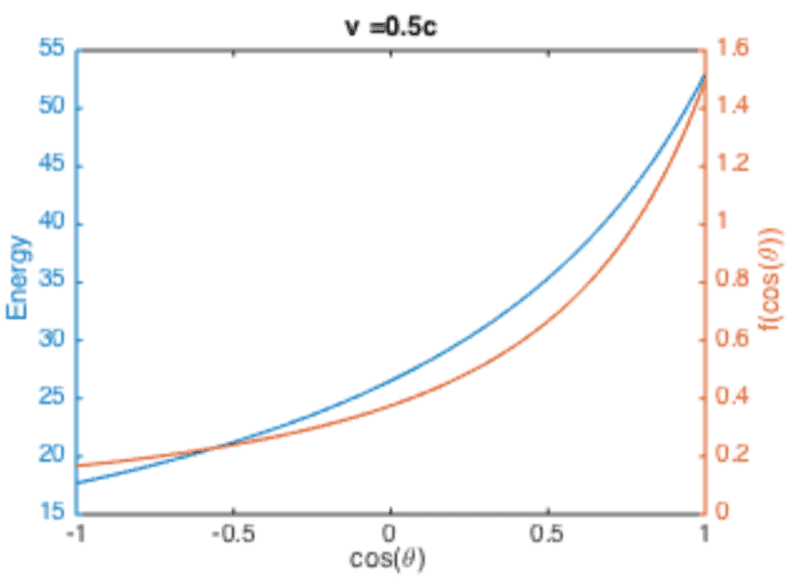The blue curve is the energy as a function of the angle ##\theta## to the forward direction and the orange curve is the distribution in the cosine of the angle.

#### Attachments

I would suggest that doing the maths is a better way to understand what is going on as you will learn why things behave as they do rather than just looking at the result on a histogram. This plot is from my SR lecture notes and shows the beaming of the neutrino resulting from a decay ##\pi^+ \to \mu^+ + \nu_\mu##, where the original pion is moving at ##v = 0.5c## (energy is in units of MeV):
View attachment 226393
The blue curve is the energy as a function of the angle ##\theta## to the forward direction and the orange curve is the distribution in the cosine of the angle.
Yes, I agree there is no point in doing a code & histogram if I don't understand at all what's happening in the situation.

Maybe it is out of context or not relevant to this, but just out of curiosity, do you know what the point of making a momentum and kinetic energy plot would be ? I understand in your plot making the energy with the angle, because then you can really see the change depending on the direction of the decay and it seems to show some pertinent information. I'm going to somehow have to include the angle in my plot anyways I assume, even though it was never explicitly asked of me....

I would suggest that doing the maths is a better way to understand what is going on as you will learn why things behave as they do rather than just looking at the result on a histogram. This plot is from my SR lecture notes and shows the beaming of the neutrino resulting from a decay ##\pi^+ \to \mu^+ + \nu_\mu##, where the original pion is moving at ##v = 0.5c## (energy is in units of MeV):
View attachment 226393
The blue curve is the energy as a function of the angle ##\theta## to the forward direction and the orange curve is the distribution in the cosine of the angle.
I guess what i'm saying is, as long as the momentum of the pion is smaller than the momentum of the lambda (which I can calculate and set as a constraint in my code) it doesn't really matter what the momentum is right ? There's no definite way of predicting it

Orodruin
Staff Emeritus
Homework Helper
Gold Member
2021 Award
You never know what the momentum will be. In the rest frame you can know what the magnitude of the momentum will be because everything is symmetric, but you do not know which direction it will go in.

You never know what the momentum will be. In the rest frame you can know what the magnitude of the momentum will be because everything is symmetric, but you do not know which direction it will go in.
Okay, it makes sense. I think I understand now. Thank you for all the help

You never know what the momentum will be. In the rest frame you can know what the magnitude of the momentum will be because everything is symmetric, but you do not know which direction it will go in.
One last quick question, if I knew the angle at which it was decaying which formula would I use to find the momentum ?

Orodruin
Staff Emeritus
Homework Helper
Gold Member
2021 Award
One last quick question, if I knew the angle at which it was decaying which formula would I use to find the momentum ?
You should be able to deduce this from 4-momentum conservation.

You should be able to deduce this from 4-momentum conservation.
I think I understand the concept of 4 momentum, but I am unsure about if I will have to be using sin/cos or some type of geometry to find the angles. I understand the conservation of momentum so then, only the x component will change and it will be the cos of the angle ?

But my problem is there are two particles decaying, the pion and the proton so they would potentially both have different angles & momentum except I only focus on the pion right now

Orodruin
Staff Emeritus
Homework Helper
Gold Member
2021 Award
I think I understand the concept of 4 momentum, but I am unsure about if I will have to be using sin/cos or some type of geometry to find the angles. I understand the conservation of momentum so then, only the x component will change and it will be the cos of the angle ?
4-momentum conservation is just energy and momentum conservation wrapped up into one and a more convenient way of constructing invariant quantities.

I suggest you write down the general momentum and energy conservation equations assuming that the pion has some unknown total momentum at some angle ##\theta## to the initial direction.

4-momentum conservation is just energy and momentum conservation wrapped up into one and a more convenient way of constructing invariant quantities.

I suggest you write down the general momentum and energy conservation equations assuming that the pion has some unknown total momentum at some angle ##\theta## to the initial direction.
In this case I would assume the the energy conservation is simply Ea=Eb+Ec

However for momentum if I separate into the three components,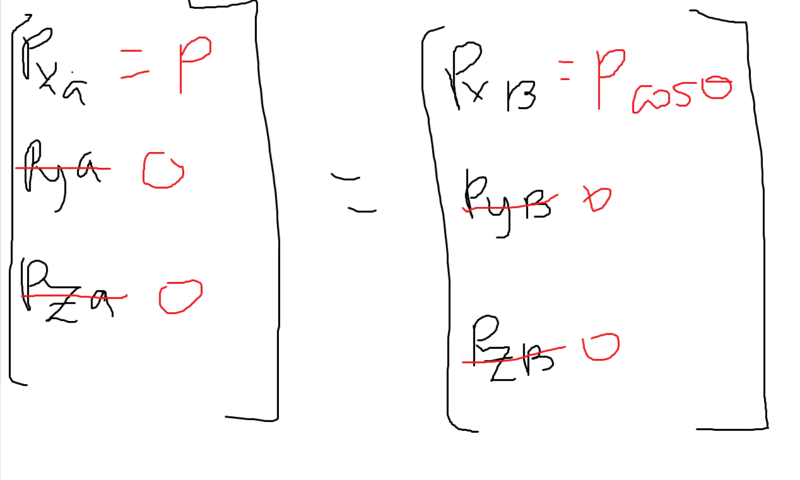but then I am ignoring the proton and if I add in that
Pa=Pb+Pc I'm not sure how to combine the angle to this

#### Attachments

4-momentum conservation is just energy and momentum conservation wrapped up into one and a more convenient way of constructing invariant quantities.

I suggest you write down the general momentum and energy conservation equations assuming that the pion has some unknown total momentum at some angle ##\theta## to the initial direction.
Alternatively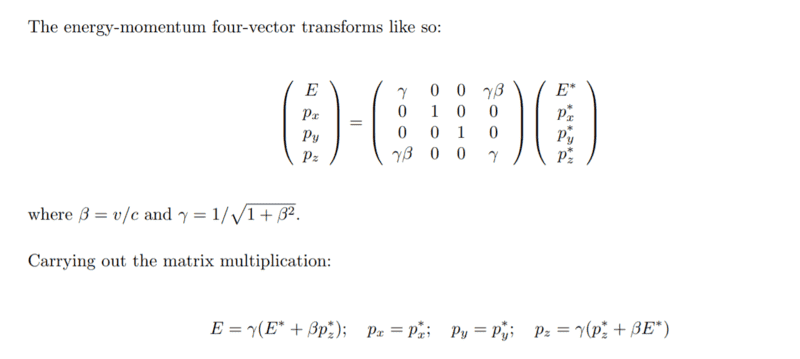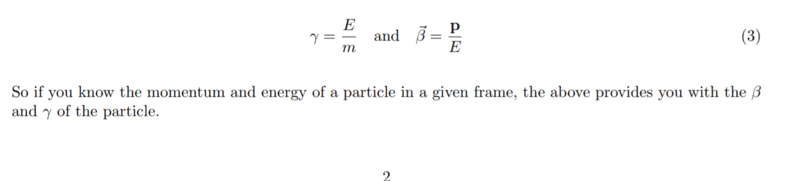http://www.dzre.com/alex/P442/lectures/lec_04.pdf

I see all their formulas for angles and such but I just can't relate it my head

Last edited: